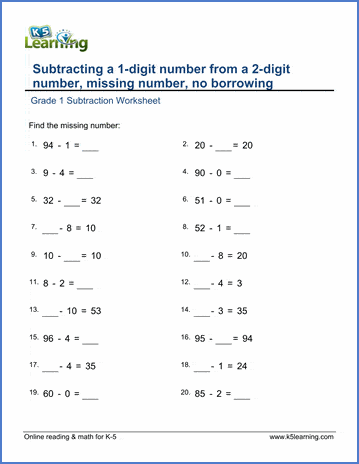# Subtraction Worksheets

## Subtraction worksheets for kindergarten through grade 5

Our free subtraction worksheets start with subtracting small numbers by counting objects and progresses to subtracting large numbers in columns.  An emphasis is placed on practicing mental subtraction so as to improve numeracy.

Kindergarten subtraction worksheets

Multi-digit subtraction: drills and practice

Subtraction flashcards

Subtraction videos

Topics include:

## Kindergarten subtraction worksheets

• Subtracting numbers within 10 by counting objects
• Counting down and subtraction
• Subtraction equations in pictures
• Add or subtracts 1s and 2s
• Subtract within 5
• Subtract single digit numbers (up to 10, 20)
• Subtract vertically
• Simple subtraction word problems

• Subtraction using objects (up to 10, 20)
• Subtract using number lines
• Subtraction sentences and number lines
• Subtracting within 0 - 10, 0-20
• Missing minuend or subtrahend (0-10)
• Subtracting a single digit number from a 2-digit number (no regrouping)
• Subtracting whole tens
• Subtracting from a whole ten
• Subtracting 1 and 2 digit numbers in columns (no regrouping)
• Mixed addition - subtraction with 3-4 single digit numbers
• Subtraction word problems
• Mixed addition and subtraction word problems

• Subtraction sentences
• Subtracting within 0-10
• Subtracting within 10-19
• Subtracting a 1-digit number from a 2-digit number (no crossing the ten)
• Subtracting a 1-digit number from a whole ten
• Subtracting a 1-digit number from a 2-digit number (some regrouping)
• Subtracting a 1-digit number from a 3-digit number (some regrouping)
• Subtracting whole tens from whole tens (0-1,000)
• Subtracting whole tens from 2 or 3 digit numbers with / without regrouping
• Subtracting whole hundreds
• Subtracting 2-digit numbers from whole hundreds
• Missing minuend or subtrahend questions
• Subtracting 2 or 3 digit numbers in columns (no regrouping)
• Subtracting 2 or 3 digit numbers in columns (with regrouping)
• Borrowing across zeros
• Fact families
• Mixed addition and subtraction of 3-4 single digit numbers
• Subtraction word problems
• Mixed addition and subtraction word problems

• Subtract 1-digit from 2-digit numbers mentally
• Subtract whole tens from 2 and 3 digit numbers
• Subtract whole hundreds from 3 digit numbers
• Subtract 2-digit numbers from 100
• Subtract whole hundreds from 3 and 4 digit numbers
• Subtracting whole hundreds from whole hundreds
• Subtracting whole tens from 1,000
• Subtracting 3-digit numbers from whole thousands
• Missing minuend / subtrahend questions
• Subtracting 3-4 digit numbers in columns
• Borrowing across 2 or 3 zeros
• Subtraction word problems

• Subtracting within 100
• Subtracting 2-digit numbers from whole hundreds
• Subtracting whole tens and whole hundreds
• Missing minuend problems
• Subtract any number from 1,000
• Subtract any 3-digit number from whole thousands
• Subtract 3, 4, 5 and 6 digit numbers in columns
• Regrouping across 2 or 3 zeros
• Mixed addition and subtraction word problems
• Mixed 4 operations word problems

• Missing minuend and subtrahend problems with large numbers
• Subtracting large numbers in columns
• Mixed 4 operations word problems

## Related topics